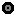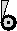#Event

## Interpretation

An event symbol represents something that happens at an instant in time, such as the arrival of a package. The event represented by a symbol may occur any number of times during a model run, or not at all. An event occurrence can have a numerical magnitude, and having a magnitude of zero is the same as not occurring. The effects of an event occurrence can be proportional to its magnitude. Alternatively it can be boolean, i.e., all-or-nothing. Event symbols should not have array equations, but can go in multi-instance submodels, in which case the event can occur independently in each instance of the submodel.

In system-dynamics models, time advances by regular steps. When an event is about to occur, Simile will execute a time step with a size that will advance the time to exactly the point at which the event is predicted to occur, in order that the effects of changes made by the event are not subject to time step rounding errors.

There are three types of event, which differ in how they are triggered. The equation dialogue for an event includes radio buttons to select between these event types, and they are distinguished by different 'decorations' on the event symbol in the model diagram.

 Modelling use Set-up of "event"Limit event : This represents an event that occurs when a model value reaches some pre-set limit. It requires an equation and a minimum and/or  maximum value to be entered. The event occurs when the value of the equation reaches the minimum or maximum, and the event should cause  the value to go no further in that direction. The event is boolean (i.e., has no magnitude) if only one limit is present. If both are present its magnitude is -1 at the lower limit and 1 at the upper limit. Hence its equation does not affect its magnitude, only its time of occurrence. The exact time at which the event occurs is determined by extrapolation of the equation value over the previous system-dynamics time step. If the integration method is Euler, linear extrapolation is used; if Runge-Kutta, it is done by a quadratic approximation. If adaptive step-size variation is selected, a check is done that the crossing actually occurs around the predicted time, and the time step is re-calculated with a new prediction if the difference is too great. Only has inflluence arrows from continuous valued components One or more influence arrows pointing from it to other events, squirts or states Magnitude is on/off if one limit supplied, integer of -1 or 1 if both limits suppliedTime series: This gets its values from outside the model like a time series variable parameter, but the event occurs at the time points entered, with the values entered giving the magnitudes of the corresponding occurrences. 'Interpolate' is not allowed (nothing happens between the time points) but 'restart' is, so a pattern of event firing can repeat. The equation of a time-series event only serves to define its units. A slider control can be generated for each such variable, and the user can generate events during the course of a simulation run by moving the slider left or right to set its magnitude and hitting the'zap' button. If a time series event symbol is put inside a multi-instance submodel, the values in the series must be arrays with the dimensions of the submodel. At each time point in the series, the event will occur in all instances of the submodel for which the corresponding array element is non-zero (or 'true' if type is boolean). No influence arrows pointing to it. One or more influence arrows pointing from it to other events, squirts or states. Occurrence times and magnitudes given by series dataDerived event (No decorations): This is an event that occurs as a result of other event occurrences. It must have an influence from at least one other event or squirt, and may occur whenever any of them occur. It may also have influences from other components. Its equation is evaluated when it is triggered, and gives its magnitude. The equation can include the special function 'trigger_magnitude()' which gives the magnitude of the triggering event, or their sum if more than one happens simultaneously. If the trigger magnitudes sum to zero, or the equation evaluates to zero (or 'false' if type is boolean) the event will not occur. The equation of a derived event can be of the form after(t,x) where t and x are subexpressions. In this case the magnitude of the event is x at the time of triggering, but it does not occur until t time units later. This is the only context in which after(...) can be used. Must have an influence from at least one other event or squirt, can have other influences to it One or more influence arrows pointing from it to other events, squirts or states.